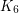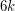Importance: Medium ✭✭
 Author(s): Ringel, G.
 Subject: Graph Theory » Coloring » » Vertex coloring
 Keywords:
 Posted by: fhavet on: March 6th, 2013
Problem   What is the maximum number of colours needed to colour countries such that no two countries sharing a common border have the same colour in the case where each country consists of one region on earth and one region on the moon ?

In term of graphs, it can be rephrased as follows. What is the maximum chromatic number of a graphwhich is the union of two planar graphs (on the same vertex set)?

If a graphonvertices is the union of two planars graphs, then it has at mostedges, and so it is has a vertex of degree at most. An easy induction shows thatis 12-colourable, as observed by Heawood [He]. Gardner [G] reported an example requiring 9 colours (the join ofand). It is not known if configurations exist requiring 10, 11, or 12 colours.

More generally, one may ask for the maximum chromatic number of the union ofplanar graphs.

Problem   What is the maximum chromatic number of a graphwhich is the union ofplanar graphs?

The same reasoning as above shows thatcolours are always sufficient. The minimum number of planar graphs to decompose a complete graph [BW] gives a lower bound of.

## Bibliography

[BW] L. W. Beineke and A. T. White, Topological Graph Theory, Selected Topics in Graph Theory, L. W. Beineke and R. J. Wilson, eds., Academic Press, 15-50, 1978.

[G] M. Gardner, Mathematical Recreations: The Coloring of Unusual Maps Leads Into Uncharted Territory. Sci. Amer. 242, 14-22, 1980.

[He] P.J. Heawood, Map Colour Theorems. Quart. J. Pure Appl. Math. 24, 332-338, 1890.

*[R] G. Ringel, Färbungsprobleme auf Flachen und Graphen. Berlin: Deutsche Verlag der Wissenschaften, 1959.

* indicates original appearance(s) of problem.